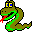#Boost C++ Libraries

...one of the most highly regarded and expertly designed C++ library projects in the world.#DFS Visitor Concept

This concept defines the visitor interface for depth_first_search(). Users can define a class with the DFS Visitor interface and pass an object of the class to depth_first_search(), thereby augmenting the actions taken during the graph search.

### Refinement of

Copy Constructible (copying a visitor should be a lightweight operation).

### Notation

 V A type that is a model of DFS Visitor. vis An object of type V. G A type that is a model of Graph. g An object of type G. e An object of type boost::graph_traits::edge_descriptor. s,u An object of type boost::graph_traits::vertex_descriptor.

none

### Valid Expressions

NameExpressionReturn TypeDescription
Initialize Vertex vis.initialize_vertex(s, g) void This is invoked on every vertex of the graph before the start of the graph search.
Start Vertex vis.start_vertex(s, g) void This is invoked on the source vertex once before the start of the search.
Discover Vertex vis.discover_vertex(u, g) void This is invoked when a vertex is encountered for the first time.
Examine Edge vis.examine_edge(e, g) void This is invoked on every out-edge of each vertex after it is discovered.
Tree Edge vis.tree_edge(e, g) void This is invoked on each edge as it becomes a member of the edges that form the search tree.
Back Edge vis.back_edge(e, g) void This is invoked on the back edges in the graph. For an undirected graph there is some ambiguity between tree edges and back edges since the edge (u,v) and (v,u) are the same edge, but both the tree_edge() and back_edge() functions will be invoked. One way to resolve this ambiguity is to record the tree edges, and then disregard the back-edges that are already marked as tree edges. An easy way to record tree edges is to record predecessors at the tree_edge event point.
Forward or Cross Edge vis.forward_or_cross_edge(e, g) void This is invoked on forward or cross edges in the graph. In an undirected graph this method is never called.
Finish Vertex vis.finish_vertex(u, g) void This is invoked on vertex u after finish_vertex has been called for all the vertices in the DFS-tree rooted at vertex u. If vertex u is a leaf in the DFS-tree, then the finish_vertex function is call on u after all the out-edges of u have been examined.

### Python

To implement a model of the DFSVisitor concept in Python, create a new class that derives from the DFSVisitor type of the graph, which will be named GraphType.DFSVisitor. The events and syntax are the same as with visitors in C++. Here is an example for the Python bgl.Graph graph type:
```class count_tree_edges_dfs_visitor(bgl.Graph.DFSVisitor):
def __init__(self, name_map):
bgl.Graph.DFSVisitor.__init__(self)
self.name_map = name_map

def tree_edge(self, e, g):
(u, v) = (g.source(e), g.target(e))
print "Tree edge ",
print self.name_map[u],
print " -> ",
print self.name_map[v]
```

 Copyright © 2000-2001 Jeremy Siek, Indiana University (jsiek@osl.iu.edu) Lie-Quan Lee, Indiana University (llee@cs.indiana.edu) Andrew Lumsdaine, Indiana University (lums@osl.iu.edu)